# Isosceles Triangle

Here we will learn about isosceles triangles, including what an isosceles triangle is and how to solve problems involving their sides and their angles.

There are also isosceles triangles worksheets based on Edexcel, AQA and OCR exam questions, along with further guidance on where to go next if you’re still stuck.

## What is an isosceles triangle?

An isosceles triangle is a type of triangle with the following properties.

• Two sides of equal length.
• Two equal angles.

The two sides that are opposite the two equal base angles are equal in length. The third side which is unequal is sometimes known as the base of the triangle.

A triangle with an obtuse angle is sometimes known as an obtuse triangle

A triangle with only acute angles is sometimes known as an acute triangle.

Properties of triangles are very important within geometry. They are one of the most common shapes to recognise for angles in parallel lines, circle theorems, interior angles, trigonometry, Pythagoras’ theorem and many more. You therefore must be familiar with their individual properties.

### What is an isosceles triangle?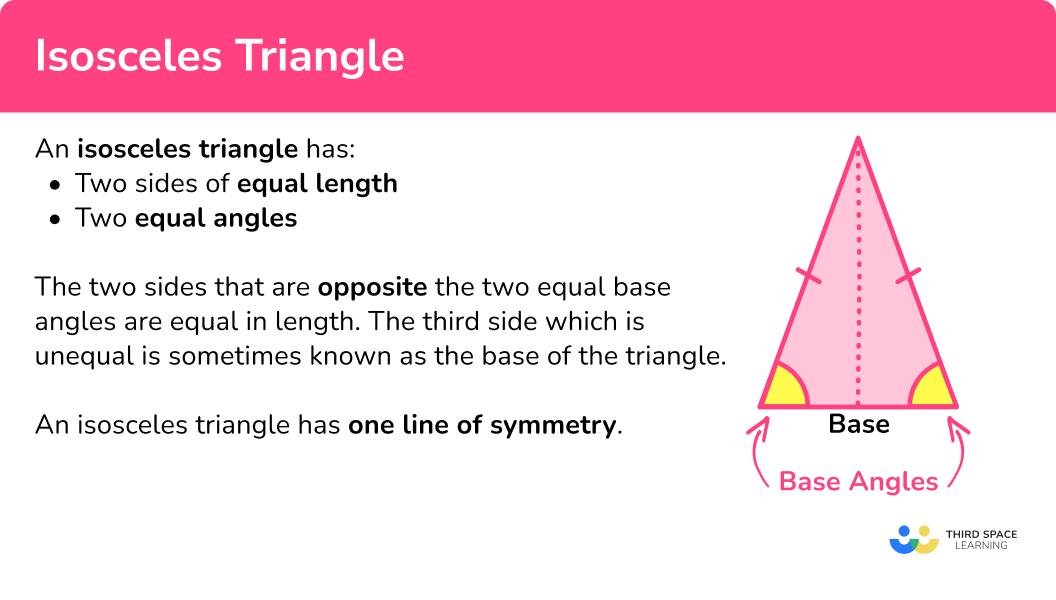### Isosceles right angled triangle

An isosceles triangle which also contains a right-angle can be known as an isosceles right triangle. This is a special case of an isosceles triangle.

The two equal angles are both 45^{\circ}.

### Symmetry

An isosceles triangle has \bf{1} line of symmetry. The line goes from the vertex to the midpoint of the side between the base angles. The line of symmetry is perpendicular to the base of the isosceles triangle. The line of symmetry is a perpendicular bisector of the base.

An isosceles triangle has order of rotational symmetry 1 as it only looks the same once in a full turn.

### Area of an isosceles triangle

The area of an isosceles triangle can be found by using the formula

A=\frac{1}{2}bh

where b is the base length and h is the perpendicular height of the triangle. Sometimes these values need to be calculated.

For example, to calculate the area of the isosceles triangle below, we need to know the value for the base b, and the value of the perpendicular height h.

Here b=1 and h=1.2. This is because these two values are perpendicular to each other. The value of 1.3 \ m is not the height of the triangle, it is one of the two equal side lengths, so beware!

Substituting the values for b and h into the area formula, we have

\begin{aligned} A&=\frac{1}{2}bh \\\\ &=\frac{1}{2}\times{1}\times{1.2} \\\\ &=0.6\text{ m}^{2} \end{aligned}

Remember that an area uses square units.

Step-by-step guide: Area of an isosceles triangle

## How to solve problems involving isosceles triangles

In order to solve problems involving isosceles triangles:

1. Locate known angles, including the pair of equal angles, and calculate any necessary unknown angles.
2. Locate known sides, including the pair of equal sides, and calculate any necessary unknown side lengths.
3. Solve the problem using any necessary values.

### Explain how to solve problems involving isosceles triangles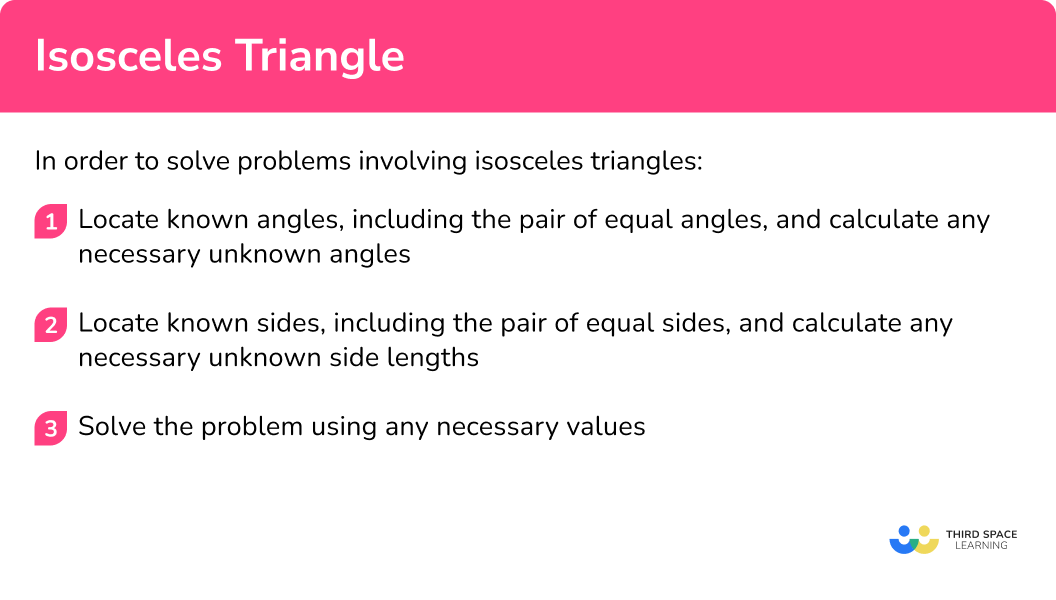### Related lessons on triangles

Isosceles triangle is part of our series of lessons to support revision on polygons and triangles. You may find it helpful to start with the main triangles lesson for a summary of what to expect, or use the step by step guides below for further detail on individual topics. Other lessons in this series include:

## Isosceles triangle examples

### Example 1: finding the missing angle in an isosceles triangle

Determine the size of angle x.

1. Locate known angles, including the pair of equal angles, and calculate any necessary unknown angles.

The pair of equal angles are at the base of this triangle as they are opposite the two equal side lengths and so the angle at B is equal to the angle at C which is 50^{\circ}.

2Locate known sides, including the pair of equal sides, and calculate any necessary unknown side lengths.

The two equal side lengths are AB and AC . We do not need to know the length of any side for this question.

3Solve the problem using any necessary values.

Using the fact that angles in a triangle add up to 180^{\circ},

180-(50+50)=80^{\circ}.

Angle x=80^{\circ}

### Example 2: finding the missing base angle

Calculate angle x.

Locate known angles, including the pair of equal angles, and calculate any necessary unknown angles.

Locate known sides, including the pair of equal sides, and calculate any necessary unknown side lengths.

Solve the problem using any necessary values.

### Example 3: finding the missing angle including exterior angles

Determine the size of the exterior angle CBD , labelled x.

Locate known angles, including the pair of equal angles, and calculate any necessary unknown angles.

Locate known sides, including the pair of equal sides, and calculate any necessary unknown side lengths.

Solve the problem using any necessary values.

### Example 4: perimeter of an isosceles triangle

The perimeter of the triangle is 24 \ cm. Find the value of y.

Locate known angles, including the pair of equal angles, and calculate any necessary unknown angles.

Locate known sides, including the pair of equal sides, and calculate any necessary unknown side lengths.

Solve the problem using any necessary values.

### Example 5: perimeter of an isosceles triangle, worded problem

The perimeter of an isosceles triangle is 35 \ cm. The length of the unequal base is half the length of the equal sides. Find the length of the base.

Locate known angles, including the pair of equal angles, and calculate any necessary unknown angles.

Locate known sides, including the pair of equal sides, and calculate any necessary unknown side lengths.

Solve the problem using any necessary values.

### Example 6: perimeter of a polygon

Here is an isosceles triangle.

Three of these triangles are put together to make a quadrilateral as shown in the diagram below.

Find the perimeter of the quadrilateral.

Locate known angles, including the pair of equal angles, and calculate any necessary unknown angles.

Locate known sides, including the pair of equal sides, and calculate any necessary unknown side lengths.

Solve the problem using any necessary values.

### Common misconceptions

• Angles in polygons

Make sure you know your angle properties. Getting these confused causes quite a few misconceptions.

◌ Angles in a triangle total 180^{\circ} .

◌ Angles in a quadrilateral total 360^{\circ} .

• Angle facts

Make sure you know your angle properties.

◌ Adjacent angles on a straight line add up to 180^{\circ} .

◌ Vertically opposite angles are equal.

◌ Alternate angles are equal.

◌ Corresponding angles are equal.

• Base angles

The base angles of an isosceles triangle are not necessarily at the bottom of the triangle.

• Sides of equal length

Make sure that you get the equal sides and angles in the correct position. It is the 2 sides which are opposite the 2 equal base angles which are equal in length.

### Practice isosceles triangle questions

1. Which of these diagrams shows an isosceles triangle?The dash marks on the sides of the triangle indicate which lengths are equal. We need the triangle which has the same mark on two sides.

2. What is the order of rotational symmetry for an isosceles triangle?

0123An isosceles triangle only looks the same once in a full turn. Therefore it has order of rotational symmetry 1.

3. Find the size of the missing angle x.72^{\circ}36^{\circ}54^{\circ}18^{\circ}The given angle is a base angle. The other base angle will also be 72^{\circ}. We need to use the fact that angles in a triangle add up to 180^{\circ}.

180-(2\times 72)=36^{\circ}

4. Find the size of the missing angle x.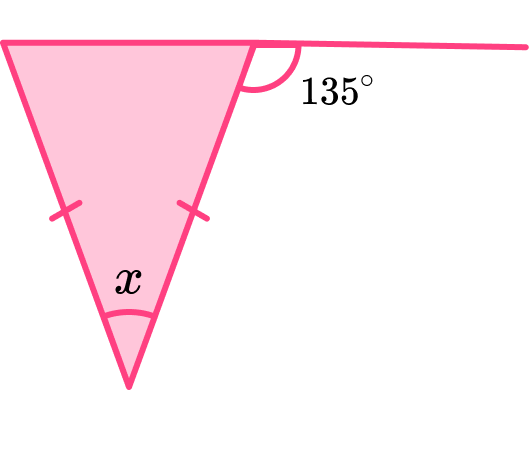45^{\circ}90^{\circ}80^{\circ}135^{\circ}The given angle is an adjacent angle on a straight line with a base angle.

180-135=45^{\circ}

The two base angles are equal.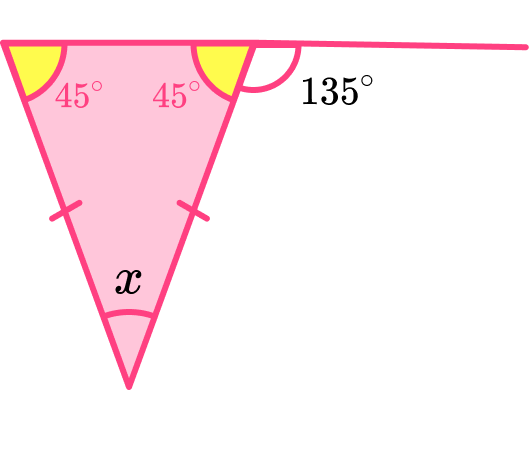The missing angle can be found as the sum of angles in a triangle is 180^{\circ}.

180-(2\times{45})=90^{\circ}

Therefore angle x=90^{\circ} .

5. Calculate the perimeter of this shape made from 4 congruent isosceles triangles.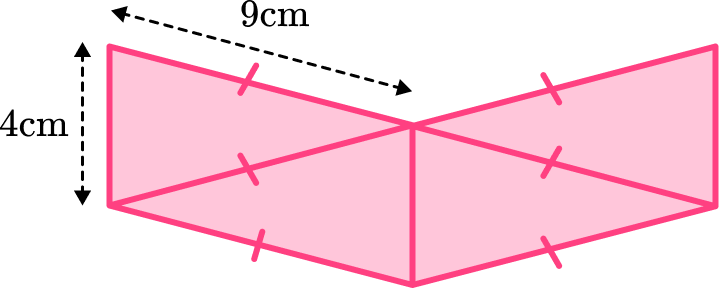13 \ cm88 \ cm44 \ cm52 \ cmAn isosceles triangle has two equal sides. Each isosceles triangle has sides 4 \ cm, \ 9 \ cm and 9 \ cm. We can add these to the diagram and then add the sides to find the perimeter.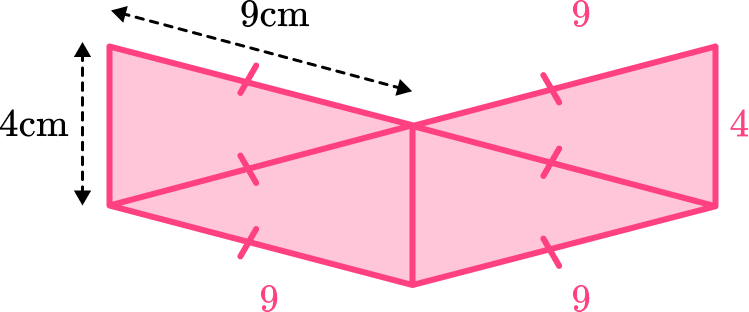4+9+9+4+9+9=44\text{ cm}

Therefore the perimeter is 44 \ cm.

6. The perimeter of an isosceles triangle is 1.2 \ m.

The ratio of the sides is 2:3:3. Find the length of the smaller base side.

45 \ m15 \ cm40 \ m30 \ cmWe can divide the perimeter by the total of the ratio parts.

1.2\div (2+3+3)=0.15

Therefore 1 part is 0.15 \ m or 15 \ cm. The base of the isosceles triangle is the unequal part which is 2 parts. Therefore the unequal base of the isosceles triangle is 30 \ cm.

2\times 15=30\text{ cm}

### Isosceles triangle GCSE questions

1. Shape A  is an isosceles triangle.Draw on the line(s) of symmetry.

(1 mark)(1)

2. (a) Here is a triangle ABC .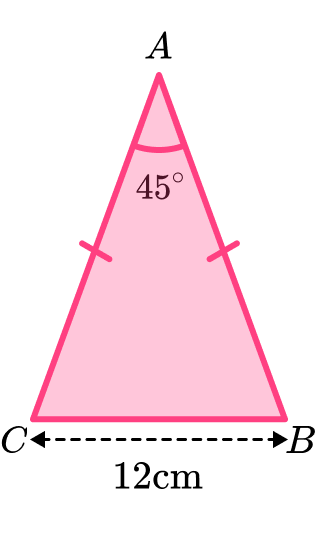Calculate angle ABC .

(b) Eight of the triangles ABC  are used to make a regular polygon.Name the regular polygon.

(c) Work out the perimeter of the regular polygon.

(5 marks)

(a)

(180-45)\div 2

(1)

67.5^{\circ}

(1)

(b) Octagon

(1)

(c)

8\times 12

(1)

96 \ cm

(1)

3. Here is a circle.

The centre of the circle is O .

A  and B  are points on the circumference.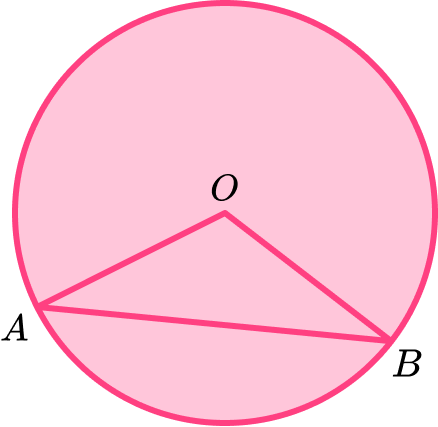Fred thinks that the triangle OAB  is an isosceles triangle.

(1 mark)

Yes. OA = OB  as they are both radii of the circle.

An isosceles triangle has two equal sides, so triangle OAB  is an isosceles triangle.

(1)

## Learning checklist

You have now learned how to:

• Recognise an isosceles triangle
• Use the properties of an isosceles triangle to solve problems

## Still stuck?

Prepare your KS4 students for maths GCSEs success with Third Space Learning. Weekly online one to one GCSE maths revision lessons delivered by expert maths tutors.

Find out more about our GCSE maths tuition programme.